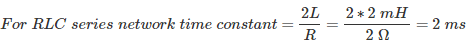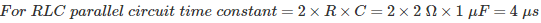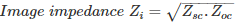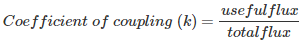# MCQs on Electric Circuits

##### Page 10 of 63. Go to page 1 2 3 4 5 6 7 8 9 10 11 12 13 14 15 16 17 18 19 20 21 22 23 24 25 26 27 28 29 30 31 32 33 34 35 36 37 38 39 40 41 42 43 44 45 46 47 48 49 50 51 52 53 54 55 56 57 58 59 60 61 62 63
01․ In RLC series circuit R = 2Ω, L = 2mH and C = 1μF. Find the time constant of the circuit?
1 μsec
2 msec
2 μsec
4 msec02․ In RLC parallel network R = 2 Ohm, L = 2mH and C = 1μF. Find the value of time constant?
2 μsec
2 msec
4 μsec
4 msec03․ Resistor stores the energy in the form of
magnetic field.
electrical field.
both A and B.
None of the above.

Resistor does not store energy in any form. It dissipates the energy in the form heat.

04․ Transients are presents in the circuit when the circuit is having
R.
L.
C.
Either L or C.

Transients are present due to storage elements. These two will not allow sudden changes of current and voltage. Therefore transients will present in the circuit due to L and C elements.

05․ In RLC series circuit R = 2Ω, L = 2mH and C = 1μF and applied voltage is 10V DC. Then what is steady state current value?
5A
2A
1A
0A

Under steady state condition inductor will act as short circuit and capacitor will act as open circuit. Therefore the circuit become open, so steady state current will be zero.

06․ Image impedance can be written by using short circuit impedance(Zsc)and open circuit impedance(Zoc) as
Zsc+Zoc
Zsc-Zoc
Zsc/Zoc
(Zsc . Zoc)½07․ In the cauer-1 form of LC network---------is series element and --------is shunt element respectively?
L and C
C and L
L and L
C and C

In the cauer-1 form represent single port network of LC filter network where L is series element and C is shunt element respectively.

08․ Poles and zeros are arranged alternatively on imaginary axis, then type of network is?
LC network
RC network
RL network
Any of the above

For LC network poles and zeros are arranged alternatively on imaginary axis.

09․ In ideal system coefficient of coupling(k) is
0
1
Both 2 and 3Therefore in ideal system useful flux is equal to total flux. Then K=1.

10․ Number of branches of a graph should be --------- number of branches of a network
greater than
less than
equals to
less than equals to

While representing of a graph, voltage sources and current sources are replaced by their internal resistance, i.e. voltage sources are by short-circuit and current sources are by open-circuit. Therefore number of branches of a graph should be ≤ to number of branches of a network.

<<<89101112>>>